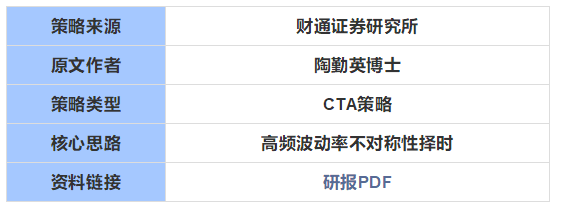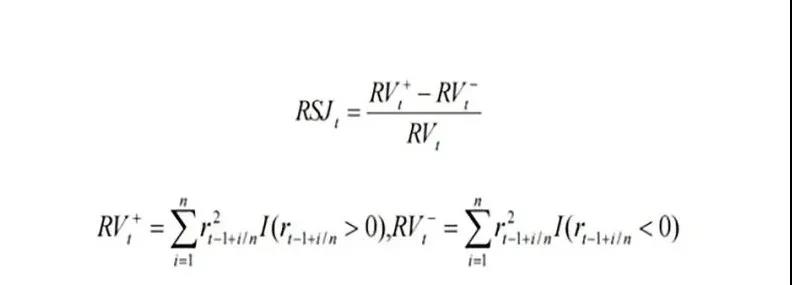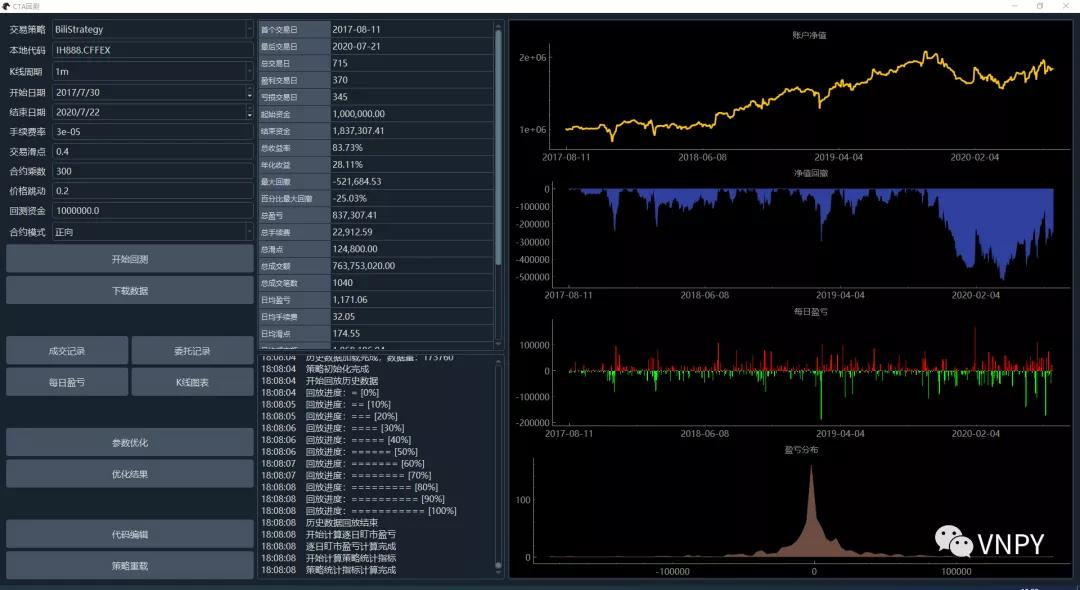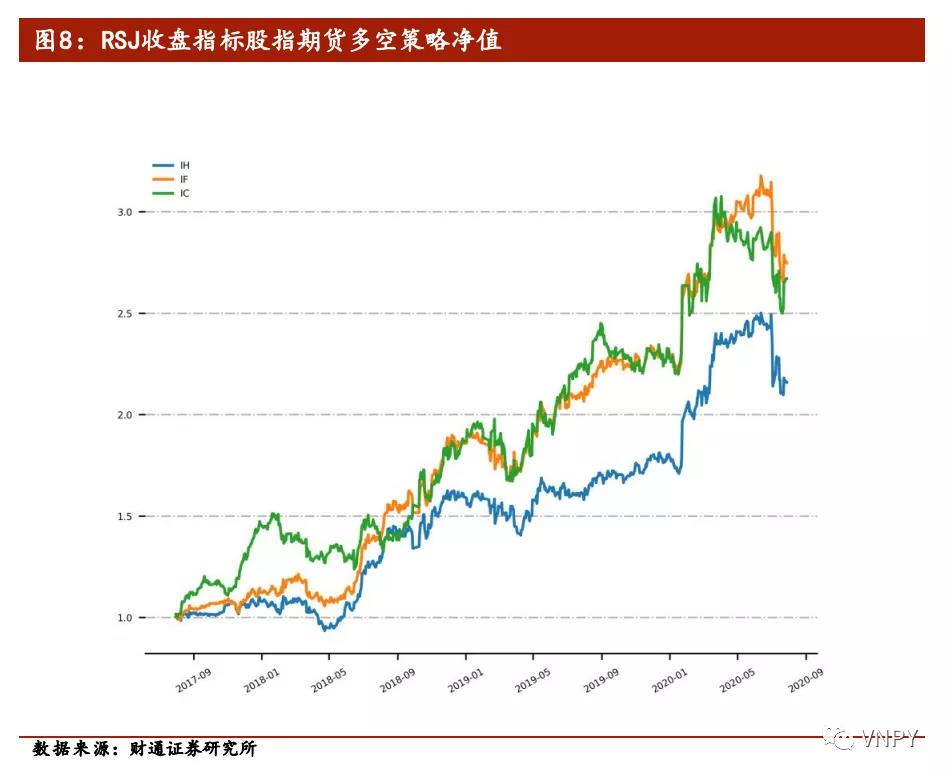vn.py官网

### 系列前言

• 券商和期货公司研究所发布的金融工程研报；
• 知名高校和业内公司发表的量化投资论文；
• 微信公众号等自媒体上分享的策略研究文章；
• 各家量化平台线上公开的经典策略源代码。

1. 找到好的量化研究资料；
2. 在vn.py中复现策略代码；
3. 和原资料对比检验正确性；
4. 加入更多灵感来改进提升。

### 基本信息### 策略原理

#### RV计算公式#### RSJ计算公式#### RSJ指标应用

• RSJ数值越大，则说明未来越倾向于下跌；
• RSJ数值越小，则说明未来越倾向于上涨。

#### 策略核心逻辑

1. 使用5分钟级别的K线来计算RSJ指标；
2. 在每日的14:55计算前一段时间（可调参数）的RSJ指标；
3. 若RSJ指标大于0则发出空头信号，反之则发出多头信号；
4. 基于指标发出的交易信号，在收盘前完成交易（比如14:56）；
5. 持仓到第二天的14:55分，然后重复2-4步骤调整仓位。

1. 策略始终在市场中（持有仓位），要么做多，要么做空；
2. 每天最多执行一次交易（如果信号方向变化）。

### 代码实现

#### 计算RSJ指标

```class NewArrayManager(ArrayManager):
def __init__(self, size=100):
""""""
super().__init__(size)
self.return_array: np.ndarray = np.zeros(size)
```

```def update_bar(self, bar: BarData) -> None:
"""更新K线"""
# 先调用父类的方法，更新K线
super().update_bar(bar)

# 计算涨跌变化
if not self.close_array[-2]:      # 如果尚未初始化上一根收盘价
last_return = 0
else:
last_return = self.close_array[-1] / self.close_array[-2] - 1

# 缓存涨跌变化
self.return_array[:-1] = self.return_array[1:]
self.return_array[-1] = last_return
```

```def rsj(self, n: int) -> float:
"""计算RSJ指标"""
# 切片出要计算用的收益率数据
return_data = self.return_array[-n:]

# 计算RV
rv = np.sum(pow(return_data, 2))

# 计算RV +/-
positive_data = np.array([r for r in return_data if r > 0])
negative_data = np.array([r for r in return_data if r <= 0])

rv_positive = np.sum(pow(positive_data, 2))
rv_negative = np.sum(pow(negative_data, 2))

# 计算RSJ
rsj = (rv_positive - rv_negative) / rv
return rsj
```

#### 交易信号执行

```def __init__(self, cta_engine, strategy_name, vt_symbol, setting):
""""""
super().__init__(cta_engine, strategy_name, vt_symbol, setting)

self.bg = BarGenerator(self.on_bar, 5, self.on_5min_bar)
self.am = NewArrayManager()
```

```def on_bar(self, bar: BarData):
"""
K线更新
"""
self.bg.update_bar(bar)
```

```def on_5min_bar(self, bar: BarData):
"""5分钟K线更新"""
# 全撤委托
self.cancel_all()

# 缓存K线
am = self.am
am.update_bar(bar)
if not am.inited:
return

# 计算技术指标
self.rsj_value = self.am.rsj(self.rsj_window)
# 判断交易信号
if bar.datetime.time() == time(14, 50):
if self.rsj_value > 0:
if self.pos > 0:
self.sell(bar.close_price - 10, 1)

self.short(bar.close_price - 10, 1)
elif self.rsj_value < 0:
if self.pos < 0:
self.cover(bar.close_price + 10, 1)

```

• vn.py中的K线采用合成时间段的开始时间戳，因此14:55分合成出的K线其时间戳（datetime）应该为14:50；
• 当发出做多信号时，策略应该持有1手的空头仓位，此时如果有昨日多头仓位，应该先卖出平仓（sell），再卖出开仓（short）。

### 回测结果

• 本地代码：IH888.CFFEX
• K线周期：1分钟
• 开始日期：2017-7-30
• 结束日期：2020-7-22
• 手续费率：0.00003
• 交易滑点：0.4
• 合约乘数：300
• 价格跳动：0.2
• 回测资金：100W• 总交易日：715
• 盈利交易日：370
• 总收益率：83.73%
• 年化收益：28.11%
• 百分比最大回撤：-25.03%

### 完整代码

Talk is cheap, show me your pnl (or code) !

```from datetime import time

import numpy as np

from vnpy.app.cta_strategy import (
CtaTemplate,
BarGenerator,
ArrayManager,
OrderData,
StopOrder)
BarData,
TickData
)

class RsjStrategy(CtaTemplate):
""""""
author = "Bili"

# 定义参数
rsj_window = 12

# 定义变量
rsj_value = 0.0

parameters = [
"rsj_window"
]
variables = [
"rsj_value"
]

def __init__(self, cta_engine, strategy_name, vt_symbol, setting):
""""""
super().__init__(cta_engine, strategy_name, vt_symbol, setting)

self.bg = BarGenerator(self.on_bar, 5, self.on_5min_bar)
self.am = NewArrayManager()

def on_init(self):
"""
策略初始化
"""
self.write_log("策略初始化")

def on_start(self):
"""
启动策略
"""
self.write_log("策略启动")
self.put_event()

def on_stop(self):
"""
策略停止
"""
self.write_log("策略停止")
self.put_event()

def on_tick(self, tick: TickData):
"""
TICK更新
"""
self.bg.update_tick(tick)

def on_bar(self, bar: BarData):
"""
K线更新
"""
self.bg.update_bar(bar)

def on_5min_bar(self, bar: BarData):
"""5分钟K线更新"""
# 全撤委托
self.cancel_all()

# 缓存K线
am = self.am
am.update_bar(bar)
if not am.inited:
return

# 计算技术指标
self.rsj_value = self.am.rsj(self.rsj_window)

# 判断交易信号
if bar.datetime.time() == time(14, 50):
if self.rsj_value > 0:
if self.pos > 0:
self.sell(bar.close_price - 10, 1)

self.short(bar.close_price - 10, 1)
elif self.rsj_value < 0:
if self.pos < 0:
self.cover(bar.close_price + 10, 1)

# 更新图形界面
self.put_event()

def on_order(self, order: OrderData):
"""
Callback of new order data update.
"""
pass

"""
Callback of new trade data update.
"""
self.put_event()

def on_stop_order(self, stop_order: StopOrder):
"""
Callback of stop order update.
"""
pass

class NewArrayManager(ArrayManager):

def __init__(self, size=100):
""""""
super().__init__(size)

self.return_array: np.ndarray = np.zeros(size)

def update_bar(self, bar: BarData) -> None:
"""更新K线"""
# 先调用父类的方法，更新K线
super().update_bar(bar)
# 计算涨跌变化
if not self.close_array[-2]:      # 如果尚未初始化上一根收盘价
last_return = 0
else:
last_return = self.close_array[-1] / self.close_array[-2] - 1

# 缓存涨跌变化
self.return_array[:-1] = self.return_array[1:]
self.return_array[-1] = last_return

def rsj(self, n: int) -> float:
"""计算RSJ指标"""
# 切片出要计算用的收益率数据
return_data = self.return_array[-n:]

# 计算RV
rv = np.sum(pow(return_data, 2))

# 计算RV +/-
positive_data = np.array([r for r in return_data if r > 0])
negative_data = np.array([r for r in return_data if r <= 0])

rv_positive = np.sum(pow(positive_data, 2))
rv_negative = np.sum(pow(negative_data, 2))

# 计算RSJ
rsj = (rv_positive - rv_negative) / rv
return rsj
```